﻿ Differences in the Mathematical Connection Capabilities of Students Taught by Using Guided Discovery Learning and Problem Based Learning Models Assisted by Autograph Viewed from Students' Numerical AbilityPublications are Open
Access in this journal
Article Versions
Export Article
• Normal Style
• MLA Style
• APA Style
• Chicago Style
Research Article
Open Access Peer-reviewed

Differences in the Mathematical Connection Capabilities of Students Taught by Using Guided Discovery Learning and Problem Based Learning Models Assisted by Autograph Viewed from Students' Numerical Ability

Friska Labora Christiani , Pargaulan Siagian, Mukhtar
American Journal of Educational Research. 2020, 8(5), 293-298. DOI: 10.12691/education-8-5-11
Received April 10, 2020; Revised May 12, 2020; Accepted May 19, 2020

Abstract

This study aims to determine (1) whether the mathematical connection ability of students taught with Autograph-assisted Guided Discovery Learning (GDL) models is higher than students taught with Autograph-assisted Problem Based Learning (PBL) models, (2) whether there is interaction between the Guided Discovery Learning (GDL) model and the Problem Based Learning (PBL) model with numerical ability on students' mathematical connection abilities. This type of research is quasi-experimental research with a population of all students of class XI Pancur Batu Private High School Methodist. This study uses two-way ANAVA. Then proceed with the Scheffe test to see whether the two groups are significantly different. Based on the statistical calculation of the Scheffe test and confirmed with SPSS, it can be concluded that (1) The ability of students' mathematical connections taught by Guided Discovery Learning (GDL) assisted by Autograph Software is higher than that of students taught with Problem Based Learning (PBL) assisted by Autograph Software, (2) There is an interaction between the learning model with numerical ability on the ability of students' mathematical connections.

1. Introduction

The ability of mathematical connections is the ability to link mathematical concepts both between mathematical learning topics and to link mathematical concepts with other fields of science as well as linking mathematical concepts with real life. According to 1 the ability of connections in mathematics plays an important role in solving mathematical problems, where the ability of connections makes students better understand mathematical problems in detail. Curriculum Standards in China in 2006 for elementary and secondary schools also emphasized the importance of mathematical connections in the form of mathematical applications, connections between mathematics and real life, and the relationship of mathematics with other subjects.

The results of the research 2 and 3 also stated the importance of increasing students 'mathematical connection ability to overcome students' difficulties in solving mathematical problems that have links with previously learned material. This is in line with the opinion 4 which states the ability of students to connect between topics in mathematics and connect mathematics with everyday problems, it is very important for students because these relationships can help students understand topics in mathematics and can create everyday problems into the mathematical model.

From some of the statements it can be concluded that the ability of mathematical connections plays an important role both in solving mathematical problems and in solving problems of everyday life. real or everyday.

There are several factors that affect the low ability of students' mathematical connections, one of which is the role of the teacher in learning activities in the classroom. For this reason, teachers need to determine learning strategies or models that can improve mathematical connection skills. The conventional method used by the teacher in class only requires students to solve problems based on formulas and examples of questions given by the teacher, not teaching students how students should solve problems. Because it is necessary to strive for learning mathematics that can improve the ability of mathematical connections. In order for these abilities to develop properly, in the mathematics learning process teachers need to provide opportunities for students to be able to improve students' abilities in developing mathematical ideas and to teach investigations in solving problems. In addition, teachers need to show the interrelationships between disciplines in mathematics.

Learning activities as described above are in accordance with the characteristics of the Problem Based Learning (PBL) model, where students are presented with real problems, focusing on interdisciplinary linkages, and authentic inquiry 5. The Problem Based Learning (PBL) model can create conditions where students can develop and maintain independent learning skills so that learning is more meaningful as indicated by critically processing learning material 6. In addition, the results of other studies indicate that the Problem Based Learning (PBL) learning model involves students directly in carrying out the stages of activities to solve problems in their own way with a variety of information or references without relying on the way the teacher solves problems so as to increase student creativity in solve problems and help students to learn new knowledge related to these problems 7.

In addition to the problem based learning (PBL) learning model, the Guided Discovery Learning model can also be a solution or an alternative in solving students' mathematical connection ability problems. Guided discovery learning is a learning activity that is designed so that students can independently discover concepts or principles through mental processes. Mental processes can observe, classify, make hypotheses, explain, measure, make conclusions and so on. The application of this learning model can stimulate students to have high curiosity so that they are more active in learning.

Some research results indicate that the guided discovery learning model can affect the improvement of students' mathematical abilities. This is shown from the results of other studies where the results of the study showed an increase in the ability to understand concepts and students' mathematical problem solving abilities 8. When students 'problem solving abilities increase, this will also affect the ability of students' mathematical connections, where when students are able to solve problems, it means students can already know the relationship between concepts that must be used in solving mathematical problems.

Each student must have different mathematical connection abilities. Many factors cause these differences, in addition to the learning model used by the teacher, students 'numerical abilities also influence students' connection abilities. According to Agustin Leoni numerical intelligence is intelligence related to numbers or mathematics. Numerical intelligence can also be interpreted as the ability to understand the relationship of numbers and solve problems related to number concepts. Furthermore, another opinion states the numerical ability is the basic ability to use numbers and the calculation process 9. Numerical mastery is also a factor influencing student learning outcomes in mathematics.

Numerical abilities are skills related to accuracy and skills in using basic arithmetic functions 10. This ability is important to master because it becomes the basis in mathematical operations. Although in the process of working on mathematical problems needed other abilities such as visual abilities, verbal abilities, connections, and so on, it is not inevitable that numerical abilities play an important and comprehensive role in working on mathematical arithmetic operations. So that every student who wants to be involved in mathematics really needs a numerical ability to solve every problem in Mathematics.

In addition to the application of learning models, teachers also need to use technology or ICT in learning mathematics. One of the effective and efficient ICTs used in learning mathematics is Autograph. Autograph is a computer program used in two-dimensional, three-dimensional learning, statistics, transformation, geometry, equations, coordinates, graphs, and quadratic equations. Autograph can enhance scientific discourse in mathematics learning that directs students to the learning experience of investigating and solving mathematical problems 11.

With the help of dynamic software combined with guided discovery learning and problem-based learning as well as good numerical ability, students are expected to be able to carry out the learning process more flexibly and students can freely try repeatedly until finally students understand the mathematical concepts learned and can connect mathematics learning in various problem solving.

2. Literature Review

2.1. Mathematical Connection Capabilities

Mathematical connection is derived from English namely Mathematical Connection which was popularized by NCTM which became the standard curriculum for elementary and secondary school mathematics learning. The ability of mathematical connections is the ability to determine the relationship of mathematical concepts with mathematical material, the relationship of mathematics with other disciplines and the relationship of mathematics with real life.

Based on the understanding of the ability of mathematical connections described previously, then in this study the indicators of mathematical connection ability are: 1) recognize the equivalent representation of the same concept, 2) recognize the mathematical procedure of a representation to the equivalent representation procedure, 3) use and assess the relationship between mathematical topics and relationships outside mathematics, 4) using mathematics in everyday life.

2.2. Problem Based Learning

Problem based learning (PBL) is a student-centered learning model and the teacher acts as a facilitator where students are confronted with mathematical problems and in groups of students conduct research, integrate theory and practice, and apply knowledge and skills to develop solutions to solve problems.

The syntax in the Problem Based Learning (PBL) model is: (1) proposing or orienting students to the problem; (2) organizing students to learn; (3) Students gather information; (4) guiding investigations individually and in groups; (5) develops and presents the work; (6) analyze and evaluate the problem solving process.

2.3. Guided Discovery Learning

Model Guided Discovery Learning (GDL) is a learning process that makes students actively find out for themselves information, investigate a problem that is guided by the teacher through supporting questions to build a mathematical concept so that students can solve a problem.

The syntax in the Guided Discovery Learning (GDL) model, namely: Introduction (Introduction), Overview (data), Data collection (data collection), Data Processing (data processing), Summary (conclusion), Assessment (assessment).

3. Research Methods

This research uses a quantitative descriptive approach with a quasi experimental method. Based on the research design that will be used, this study aims to examine the ability of students' mathematical mathematical connections affected by autograph-assisted Problem Based Learning (PBL) models and autograph-assisted Guided Discovery Learning models. The design of this research is Post Test Only Control Design. The experimental design used is factorial design. The factorial design used is a 2 x 3 factorial design, as in Table 1 below:

4. Research Results

The data analyzed are tests of students 'numerical ability and tests of students' mathematical ability. Then the test analysis is done by two-way analysis of variance (ANAVA). The test results provide information about students' mathematical connection skills after being taught with the Autograph-assisted Guided Discovery Learning model in Experiment I class and students who are taught with the Autograph-assisted Problem Based Learning model in Experiment II class.

4.1. Student Numerical Ability Data Results

Numerical ability tests are given to students to find out the types of numerical abilities especially mathematical skills students have. The numerical ability grouping of students (high, medium, and low) is formed based on the classification criteria of numerical ability of students. The results of the grouping are presented in the following Table 2:

Based on Table 2 above obtained in the GDL class the numerical ability of students for the medium category there are 5 students, the medium category is 15 students and the high category is 15 students. Whereas in the PBL class the level of numerical ability of students for the medium category was 5 students, the medium category was 24 students and the high category was 6 students. Furthermore, to see whether the connection capability of the GDL and PBL classes is the same or not, a statistical test is carried out, namely the normality test, homogeneity test, and two-way ANAVA.

The normality test was carried out using the Kolmogorov-Smirnov one sample test statistic in the SPSS Statistics 20.00 program with a confidence level of 95%. In this study, the homogeneity test was performed using the Levene test in the SPSS program.

4.2. Post-Test Results for Students' Mathematical Connection Ability

After the two classes are given learning by using the Guided Discovery Learning model in the experimental class I and the Problem Based Larning model in the experimental class II, then the end of the learning of the two classes is given a post-test to see the development of students' mathematical connection ability after the learning model is applied. Description of the results of the post-test mathematical connection ability of students calculated the lowest value, the highest value, and the average, and the standard deviation of each experimental class. The following are descriptive statistics from the post-test experimental class I and experimental class II presented in the following table.

From Table 3 above, it can be seen that the average post-test mathematical connection ability of students in both experimental I and experimental II classes is different. Next it needs to be tested whether the post-test connection capability of the GDL class and the PBL class are the same or not. To test the hypothesis, first test the normality and homogeneity of the post-test. Based on the Kolmogrov-Smirnov test using SPSS, it was concluded that the results of students' mathematical connection abilities in the GDL class and PBL class were normally distributed. Furthermore, the Levene test concluded that the GDL class and PBL class had homogeneous variances.

4.3. Hypothesis Testing

The purpose of data analysis to be done with inferential statistics is to test the research hypothesis. Testing the statistical hypothesis in this study is the two-way Anava using SPSS version 20.00. The linear model for this research is: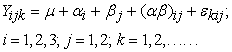Keterangan: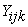is a score of the connection ability of the k-student, in the i- numerical ability which gets j- learning.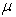is the average score of a student's actual connection ability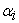is the additive effect of i-numeric ability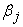is the additive effect of the j-the learning model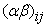is the interaction effect of the-i the numerical ability and the j- learning model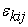is the effect of experimental deviations from k- the student scores, on the i- numerical ability, which get j- learning model.

4.3.1. Hypothesis Test I

After the test requirements are met then the next hypothesis test is done using two-way ANAVA. The first hypothesis that will be tested in this study is to test differences in the ability of students' mathematical connections taught by the Guided Discovery Learning model and students who are taught with the Problem Based Learning model assisted by Autograph Software. The test criterion is H0 if sig. < 0,05. Tests conducted based on Hypothesis I, namely: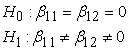Information :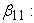The influence of Autograph-assisted Guided Discovery Learning models on the ability of students' mathematical connections.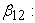The influence of Autograph-aided Problem Based Learning models on students' mathematical connection abilities.

The results of two-way ANAVA test calculations to test Hypothesis I, were confirmed by SPSS 20 statistics with sig values. amounted to 0.019. Because the value of sig. < 0.05, then H0 is rejected. Means there is an influence of learning models on students' mathematical connection ability. In other words, there is a significant difference in the ability of mathematical connections between the Guided Discoveri Learning model and the Problem Based Learning model.

4.3.2. Hypothesis Test II

Hypothesis II that will be tested in this study is that there is an interaction between the learning model with numerical ability on students' mathematical connection ability. Statistically the hypothesis can be formulated: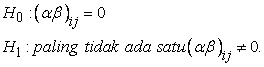Information: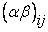is the interaction between learning models with numerical abilities on students' mathematical connection abilities.

The results of two-way ANAVA test calculations to test Hypothesis II, were confirmed by SPSS 20 statistics with sig values. of 0.039. Because the value of sig. < 0.05, then H0 is rejected, this shows the influence of interaction between the learning model with numerical ability on the ability of students' mathematical connections.

4.3.3. Scheffe Test of Mathematical Connection Ability

After conducting the two-way ANAVA test, then further tests are performed with the Scheffe method, namely comparison between rows, between columns and between cells. The summary of the results of the average calculation between cells of students' mathematical connection ability is presented in Table 4.

a. Comparative test between rows with the results of the comparative summary in the following Table 4

Based on Table 4 above, it can be concluded that in the hypothesis test µS vs µR, µT vs µR, and µT vs µS H0 are rejected, it can be concluded that there are significant differences in mathematical connection ability between students who have moderate and low numerical abilities, students who have high and low numerical ability, and students who have high and medium numerical ability.

b. Comparative test between columns with the computational summary results in the following Table 5

Based on Table 5 above, it can be concluded that the hypothesis test µG vs µP H0 is rejected, so it can be concluded that there are significant differences in mathematical connection ability between students taught with the GDL model and PBL models. Based on Table 3 above, the marginal average for the GDL learning model is 81.057, while for the PBL learning model is 72.8, so it can be concluded that the mathematical connection ability of students taught with the Guided Discovery Learning (GDL) model is higher than students taught with the Problem model Based Learning (PBL).

From Table 6 we can see the Sig value in the types of numerical ability Low, medium and high. If the Sig value < 0.05, then accept H1, which means there is a difference in the average connection ability between the Guided Discovery Learning model and the Problem Based Learning model for each type of numerical ability. From this table we can look at the analysis output with multiple comparisons. An asterisk on the mean difference or Sig. which is smaller than alpha (5%), it appears that there are differences in the ability of connections between students who are taught with the Guided Discovery Learning model and students who are taught with the Problem Based Learning model in each type of numerical ability.

5. Discussion

5.1. Differences in the Connection Capabilities of Students Taught by the Guided Discovery Learning Model and Students Taught by a Problem Based Learning Model

Based on the results of data analysis, the average post-test score of the GDL class was 81.057 while in the PBL class the average post-test score was 72.8. This shows that the average post-test scores in the GDL class are higher than the PBL class. This is because that in learning GDL, teachers provide more opportunities for students to think, search for information both from books and the internet and discover for themselves a concept that is used to solve the problem presented, then students process data that has been collected through discussing the problem with group of friends.

Judging from the connection ability indicator, for each average score of the mathematical connection ability indicator students of the GDL class in the first indicator is 79 while for the PBL class is 74. For the second indicator the GDL class is worth 85 while in the PBL class is worth 76, the same for the third indicator of the GDL class is worth 78 and the PBL class is worth 70. While for the fourth indicator in the GDL class the average value of students is 77 while in the PBL class is worth 70. Thus it can be seen that the indicator of mathematical connection ability that is best achieved by students is to recognize mathematical procedure of an equivalent representation.

Based on the results of inferential statistical analysis with Anava two paths shows that there are significant differences in the ability of mathematical connections between students taught with the GDL model and PBL models. Where the mathematical connection ability of students taught by the GDL model is higher than that of students taught by the PBL model. This is caused by several things, one of which is the characteristic of the GDL model, which provides an opportunity for students to think of making a picture of the problem formulation, gathering information (data), processing data until finally making conclusions. In addition, in learning the GDL model, students with low, medium and high numerical abilities can learn together well. Students with moderate and high numerical ability are not too difficult to follow the existing learning process. With the guidance of the teacher they can collaborate with friends.

The cooperative learning model is in line with underlying learning theories such as constructivism learning theory. Basically, the approach of the theory of constructivism requires students to find themselves and transform information, check new information with old rules. So students succeed in building their own knowledge in their minds. According to Trianto cooperative learning is ruled by the theory of constructivism. This learning arises from the concept that students more easily understand and find if among students discuss each other 12.

Based on the results of the study described earlier, that the cooperative learning model can have a positive influence on the problem solving process and on the ability of students' mathematical connections. This reinforces the results of previous studies that also use cooperative learning models to improve other mathematical abilities. Among other research results states that learning using guided discovery learning models with video learning assistance is more effective than learning with conventional models 13.

Other research results state that the Problem Based Learning model is better and even more recommended than the Guided Discovery Learning model 14. However, this study found different things. Where the results of the study show that the mathematical connection ability of students taught with the Guided Discovery Learning model is higher than students taught with the Problem Based Learning model.

5.2. Interactions between Learning Models and Numerical Abilities of Mathematical Connection Capabilities

Interation is the collaboration of two independent variables or also more influential on the dependent variable. In this case, what is investigated is the collaboration between the learning model and numerical ability on students' mathematical connection abilities. Based on the results of descriptive analysis, the average value of students' mathematical connection ability in the GDL class in the category of low numerical ability was 66.4, medium numerical ability was 81.87, and High numerical ability was 85.07. While the average value of the ability of students in PBL class connections in the category of low numerical abilities was 56.6 while numerical abilities were 72.28, and numerical abilities were High 87.33. This shows the ability of students' mathematical connections for all categories of numerical abilities in the GDL class is higher than in the PBL class.

Based on the results of statistical analysis with two-way ANAVA shows the significant value of interaction between numerical ability and the model is 0.039, where the value of sig. 0.039 is smaller than 0.05 so H0 is rejected. This means that there is a significant interaction between the learning model and numerical ability on the ability of students' mathematical connections. In other words, the average difference in tests of mathematical connection ability between students in the low, medium, high category taught by the GDL model and the PBL model is significantly different. This shows that there is a joint influence that is given by the learning approach (GDL and PBL model) with the numerical ability (low, medium, hight) of students towards mathematical connection ability.

The same thing as 15 who stated that there was an interaction between the learning model and the level of students' numerical ability on learning achievement. In as 16 results show that there is an interaction between learning models with numerical ability on mathematical logic intelligence.

6. Conclusion

The conclusions in this study after analyzing the data are as follows:

1. Mathematical connection ability of students taught by the Guided Discovery Learning model assisted by Autograph software is higher than students taught by the Problem Based Learning model assisted by Autograph software.

2. There is an interaction between learning models with numerical ability on students' mathematical connection abilities.

References

  Nugraha, A. A. Analysis of Mathematical Connection Capabilities of Junior High School Students on Material for Two Variable Linear Equation Systems (SPLDV). Suska Journal of Mathematics Education, 2018, Vol. 4, No.1. In article View Article  Herawati, L. Improving Students' Mathematical Connection Capabilities Using Problem Based Learning (PBL) Models with Assisted Geogebra Software. Journal of Mathematics Teaching and Research Research, 2017.Vol.3, No.1. In article  Kurniawan, A.Y., & Kartono, S. Improving Students' Mathematical Connection Ability Through PBL Model Based on Constructivistic Material of Class X SPLDV. PRISMA Journal 1, 2018. In article  Ni'mah, A. F., Setiawani, S., & Oktavianingtyas, E. The Analysis of Mathematic Connection Capability Grade IX A of MTs Negeri 1 Jember Subchapter Cube and Block. Journal of Education, 2017, Vol.4, No.1. In article View Article  Trianto. Designing Innovative-Progressive Learning Models. Jakarta: Kencana Perdana Media Group, 2009. In article  Malan, S. B., & Ndlovu, M. Introducing Problem-Based Learning (PBL) Into a Foundation Program for Developing Self-Directed Learning Skills. South African Journal of Education, 2014, Vol. 34, No.1. In article View Article  Yustianingsih, R., Syarifuddin, H., & Yerizon. Development of Mathematical Learning Tools Based on Problem Based Learning (PBL) to Improve the Problem Solving Ability of Class VIII Students. National Journal of Mathematics Education, 2017, Vol. 1, No.2. In article  Ramadhani, R. Increased Ability to Understand Concepts and Ability to Solve Mathematical Problems of High School Students Through Guided Discovery Learning with Autograph Assistance. Journal of Education and Community Empowerment (JPPM), 2017, Vol.10, No.2. In article  Rezawatimar., Maidiyah, E., Suryawati. Numerical Ability and Its Relationship with Mathematics Learning Outcomes of Class VII Students of SMP Negeri 1 Manggeng, ABDYA in 2016/217. Scientific Journal of Mathematics Education Students. 2018, Vol.3, No.2. In article  Irawan, A. Role of Numerical and Verbal Ability in Critical Thinking in Mathematics at the High School Level. Formative Journal, 2016, Vol. 6, No.2. In article  Ramadhani, R. Increased Ability to Understand Concepts and Ability to Solve Mathematics Problems of High School Students Through Guided Discovery Learning with Autograph Assistance. Journal of Education and Community Empowerment (JPPM), 2017, Vol.10, No.2. In article  Trianto. Designing Innovative-Progressive Learning Models. Jakarta: Kencana Perdana Media Group. 2009 In article  Ulfa, K., Buchori, A., & Murtianto, Y. H. Effectiveness of Guided Discovery Learning Models for Learning Videos in Knowing Differences in Students' Mathematical Problem Solving Ability. Journal of Mathematics Education, Science and Technology. 2017, Vol.2, No.2. In article View Article  Yuliasari, E. Eksperimentasi Model PBL dan Model GDL Terhadap Kemampuan Pemecahan Masalah Matematis Ditinjau dari Kemandirian Belajar. Jurnal Ilmiah Pendidikan Matematika. 2017, Vol. 6, No.1. In article View Article  Nurcahyono. Ekperimentasi Model Pembelajaran Kooperatif Tipe TPS dan NHT dengan Pendekatan CTL pada Materi Pecahan Ditinjau dari Tingkat Intelegensi Siswa Kelas V SD Sekecamatan Banyuurip Kabupaten Purworejo. 2013, Vol 8, No.2. In article  Dudung, A. The Effect of Learning Models and Numerical Abilities on Learning Outcomes Machinery by Controlling Engineering Potential. Journal of Educational Technology 2017, Vol 19, No.3. In article

Published with license by Science and Education Publishing, Copyright © 2020 Friska Labora Christiani, Pargaulan Siagian and MukhtarThis work is licensed under a Creative Commons Attribution 4.0 International License. To view a copy of this license, visit http://creativecommons.org/licenses/by/4.0/